# Ray diagrams for converging (convex) lens

If an object is on one side of the convex lens, the convex lens can form the image of the object. If the position of the object on one side of the convex lens is known, how to draw the image formation of the object? Suppose an object is on the left side of the convex lens, as shown below.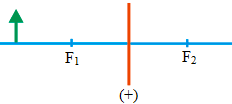Orange line = convex lens

Blue line = principal axis

Arrow (green) = object

F1 = focal length 1 and F2 = focal length 2

The figure of the object’s image is obtained by drawing all the beams of light passing through the object, but this is less practical because there will be many lines representing light beams. For simplicity, just a few beams of light are chosen to represent all the beams of light passing through the object. Since this event involves refraction of light, the law of refraction of light must be obeyed when drawing the image formation.

Three beams of light or three rays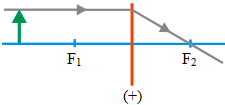Ray 1 :

Ray 1 or the light beam 1 that comes towards the convex lens are drawn parallel to the principal axis of the lens and touch the upper end of the object,

then refracted by the convex lens, where the refracted beam of light must pass through the focal point 2. The incoming rays and refractive rays that are drawn must fulfill the law of refraction of light.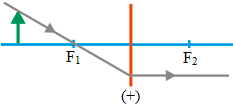Ray 2 :

Ray 2 or the light beam 2 that come to the convex lens are drawn to pass the focal point 1 and touch the upper end of the object,

then refracted by the convex lens, where the refracted beam of light must parallel the principal axis. The incoming rays and the refractive rays that are drawn must fulfill the law of refraction of light.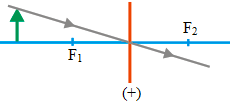Ray 3 :

The ray 3 or the light beam 2 that come to the convex lens are drawn to pass through the point of intersection of the convex lens and the principal axis and touch the upper end of the object; then the light beam is forwarded.

The formation of the image (two rays)

The image formation can be drawn using only two rays, as shown below. If using two rays, there are three possible images formation.

Ray 1 and 2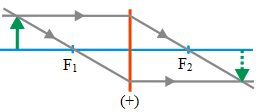Ray 1 and 3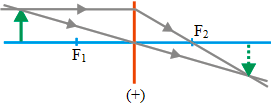Ray 2 and 3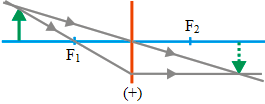The figure the image formation using only two beams of light needs to be also adjusted by the object distance from the convex lens. If the object distance from the convex lens is as in the figure above, there are three ways to draw the image formation using only two rays. If the object distance from the convex lens is different from the figure above, for example, the object is between the focal point and the center of curvature,

then there is no need for three ways to draw the image formation, maybe there are only two ways to draw.

If you draw an image formation by the convex lens, you can choose one method.

The formation of the image (three rays)

The object formation can be drawn using three rays, as shown below.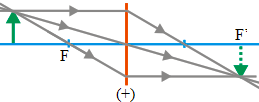If the object distance from the convex lens is not like the figure above, for example, the object is between the convex lens and the focal point, or an object is between the focal point and the center of the curvature of the convex lens, then it is no need to draw the image formation using three ways like the figure above. There can only be two ways to draw the image formation.